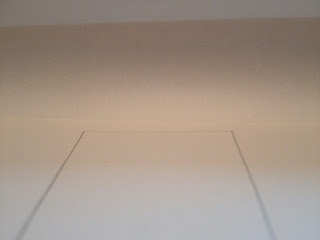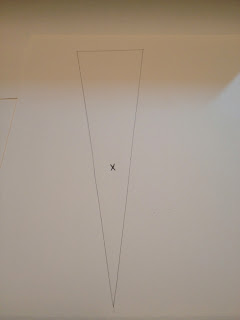### Software Rasterizer Part 1

Introduction
Software rasterizer can be used for occlusion culling, some games such as Killzone 3 use this to cull objects.  So I decided to write one by myself. The steps are first to transform vertices to homogenous coordinates, clip the triangles to the viewport and then fill the triangles with interpolated parameters.  Note that the clipping process should be done in homogenous coordinates before the perspective division, otherwise lots of the extra work are need to clip the triangles properly and this post will explain why clipping should be done before the perspective division.

Points in Homogenous coordinates
In our usual Cartesian Coordinate system, we can represent any points in 3D space in the form of (X, Y, Z). While in Homogenous coordinates, a redundant component w is added which resulting in a form of (x, y, z, w). Multiplying any constant (except zero) to that 4-components vector is still representing the same point in homogenous coordinates. To convert a homogenous point back to our usual Cartesian Coordinate, we would multiply a point in homogenous coordinates so that the w component is equals to one:

(xyzw) -> (x/y/z/w, 1) -> (XYZ)

In the following figure, we consider the x-w plane, a point (xyzw) is transformed back to the usual Cartesian Coordinates (XYZ) by projecting onto the w=1 plane:figure 1. projecting point to w=1 plane

The interesting point comes when the w component is equals to zero. Imagine the w component is getting smaller and smaller, approaching zero, the coordinates of point (x/y/z/w, 1) will getting larger and larger. When w is equals to zero, we can represent a point at infinity.

Line Segments in Homogenous coordinates
In Homogenous coordinates, we still can represent a line segment between two points P0= (x0y0z0w0) and  P1= (x1y1z1w1) in parametric form:

L= P0 + t * (P1-P0),   where t is within [0, 1]

Then we can get a line having the shape:figure 2. internal line segment
The projected line on w=1 is called internal line segment in the above case.
But what if the coordinates of P0 and P1 having the coordinates where w0 < 0 and w1 > 0 ?figure 3. external line segment
In this case, it will result in the above figure, forming an external line segment. It is because the homogenous line segment have the form L= P0 + t * (P1-P0), when moving the parameter from t=0 to t= 1, since w0 < 0 and w1 > 0, there exist a point on the homogenous line where w=0. This point is at infinity when projected to the w=1 plane, resulting the projected line segment joining P0 and P1 passes through the point at infinity, forming an external line segment.

The figure below shows how points are transformed before and after perspective projection and divided by w:figure 4. region mapping
The blue line shows the viewing frustum, nothing unusual for the region in front of the eye. The unusual things are the points behind the eye. After perspective transformation and projected to w=1 plane, those points are transformed in front of the eye too. So for line segment with one point in front of the eye and the other behind the eye, it would be transformed to the external line segment after the perspective division.

Triangles in Homogenous coordinates
In the last section, we know that there are internal and external line segments after the perspective division, we also have internal and external triangles. The internal triangles are the one that we usually sees. The external triangles must be formed by 1 internal line segment and 2 external line segments:figure 5. external triangle
In the above figure, the shaded area represents the external triangle formed by the points P0, P1 and P2. This kind of external triangles may appear after the perspective projection transform. And this happens in our real world too:an external triangle in real worldthe full triangle of the left photo
In the left photo, it shows an external triangle with one of the triangle vertex far behind the camera while the right photo shows the full view of the triangle and the cross marked the position of the camera where the left photo is taken.

Triangles clipping
To avoid the case of external triangles, lines/triangles should be clipped in homogenous coordinates before divided by the w-component. The homogenous point (xyzw) will be tested with the following inequalities:

(-<= <= w) &&   ------ inequality. 1
(-<= <= w) &&   ------ inequality. 2
(-<= <= w ) &&   ------ inequality. 3
> 0    ------ inequality. 4

(The z clipping plane inequality is 0 <= <= w in the case for D3D, it depends on how the normalized device coordinates are defined.) Clipping by inequality 1,2,3 will effectively clip all points that with < 0 because if < 0, say = -3:

3 <= x <= -3     =>     3 <= -3

which is impossible. But the point (0, 0, 0, 0) is still satisfy the first 3 inequalities and forming external cases, so inequality 4 is added. Consider a homogenous line with one end as (0, 0, 0, 0), it will equals to:

L= (0, 0, 0, 0) + t * [ (x, y, z, w) - (0, 0, 0, 0) ] = t * (xyzw)

which represent only a single point in homogenous coordinates. So triangle (after clipped by inequality 1, 2, 3) having one or two vertices with w=0 will result in either a line or a point which can be discarded. Hence, after clipping, no external triangles will be produced when dividing by w-component. To clip a triangle against a plane, the triangle may result in either  1 or 2 triangles depends on whether there are 1 or 2 vertex outside the clipping plane:figure 6. clipping internal triangles
Then the clipped triangles can be passed to the next stage to be rasterized either by a scan line algorithm or by a half-space algorithm.

Below is the clipping result of an external triangles with 1 vertex behind the camera.clipping external triangle in software rasterizer
Below is another rasterized result:rasterized duck modelreference of the duck model
Conclusion
In this post, the maths behind the clipping of triangles are explained. Clipping should be done before projecting the homogenous point to the w=1 to avoid taking special cares to clip the external triangles. In the next post, I will talk about the perspective interpolation and the source code will be given in the next post (written in  javascript, drawing to html canvas).

And lastly special thanks to Fabian Giesen for giving feedback during the draft of this post.

References
 http://research.microsoft.com/pubs/73937/p245-blinn.pdf
 http://medialab.di.unipi.it/web/IUM/Waterloo/node51.html
 http://kriscg.blogspot.com/2010/09/software-occlusion-culling.html
 http://www.slideshare.net/guerrillagames/practical-occlusion-culling-in-killzone-3
 http://www.slideshare.net/repii/parallel-graphics-in-frostbite-current-future-siggraph-2009-1860503
 http://fgiesen.wordpress.com/2011/07/05/a-trip-through-the-graphics-pipeline-2011-part-5/
 http://devmaster.net/forums/topic/1145-advanced-rasterization/